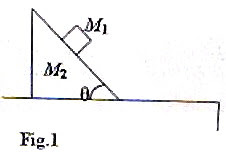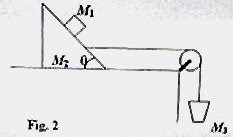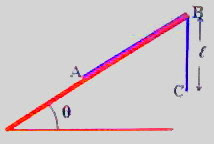## Pages

`“Life is like riding a bicycle.  To keep your balance you must keep moving.”–Albert Einstein`

## Friday, October 24, 2008

### Free Response Questions (for practice) on Newton’s Laws for AP Physics B & C

Today I give you two AP Physics practice questions on Newton’s laws of motion:(1) Free response question for AP Physics B:

Fig. 1 shows a small block of mass M1 placed on the rough inclined surface of a large wedge shaped block of mass M2 which is at rest on a rough horizontal table top. The coefficients of static friction and kinetic friction (between M1 and M2) are respectively μs and μk. Now answer the following questions (a), (b) and (c):

(a) Block M1 is just going to slide down. Draw the free body diagram for block M1 showing and identifying all the forces acting on it. (Represent M1 by a dot).

(b) What is the magnitude of the frictional force on M1 if it is at rest and does not show any sign of sliding down? Justify your answer.

(c) What is the magnitude of the frictional force on M1 if the mass M1 is sliding down? Justify your answer.Now suppose that there is no friction between the blocks M1 and M2 as well as between M2 and the table top. The system of masses M1 and M2 is connected to another mass M3 by a light inextensible string passing over a frictionless pulley of negligible mass as shown in fig.2. The mass M3 is such that the block M1 is prevented from sliding down along the block M2. Now answer the following questions (d) and (e):

(d) Determine the common acceleration of M1 and M2.

(e) Show that the condition for the block M1 not sliding along the block M2 is

M3 = (M1+M2)/(cot θ – 1)

The above question carries 15 points and you have about 17 minutes for answering it.(2) Free response question for AP Physics C:

The adjoining figure shows a uniform chain of length L placed on a rough inclined plane of angle θ with a portion of length (of the chain) overhanging from the top edge B of the incline. The coefficient of friction between the chain and the inclined plane is μ. Now answer the following questions (a), (b) and (c):

(a) In the condition shown in the figure, the chain is just going to slide up the incline. Obtain an expression for the overhanging length of the chain in terms of the given parameters.

(b) If the chain were just going to slide down the incline, will the value of the overhanging length of the chain be different from the value obtained in part (a)?Put a tick (√) mark against the correct option among (i), (ii) and (iii) given below:

(i) Yes_____ (ii) No_____ (iii) The answer will depend on the angle of the plane.

(c) If there is no friction between the chain and the inclined plane, determine the value of the overhanging length of the chain that will make it just start sliding down the plane.

The chain is now placed on a frictionless horizontal table of height H so that the portion AB of the chain is straight and is on the table (fig.) where as the remaining portion BC of length H is overhanging, with the end C just touching the ground. Now answer the following question (d) and (e):

(d) When the chain is let free it slides along the table. Take the point A (initial position of the end of the chain) as the origin and write a differential equation for the acceleration of the chain at any instant during its motion.

(e) Solve the differential equation obtained in part (d) and determine the velocity of the chain when the end A just leaves the edge of the table.

The above question carries 15 points and you are supposed to answer it in about 15 minutes.

AP Physics C aspirants should try to answer both questions. AP Physics B aspirants may omit part (e) of question No.2.

Try to answer these questions. I’ll be back soon with model answers for you.